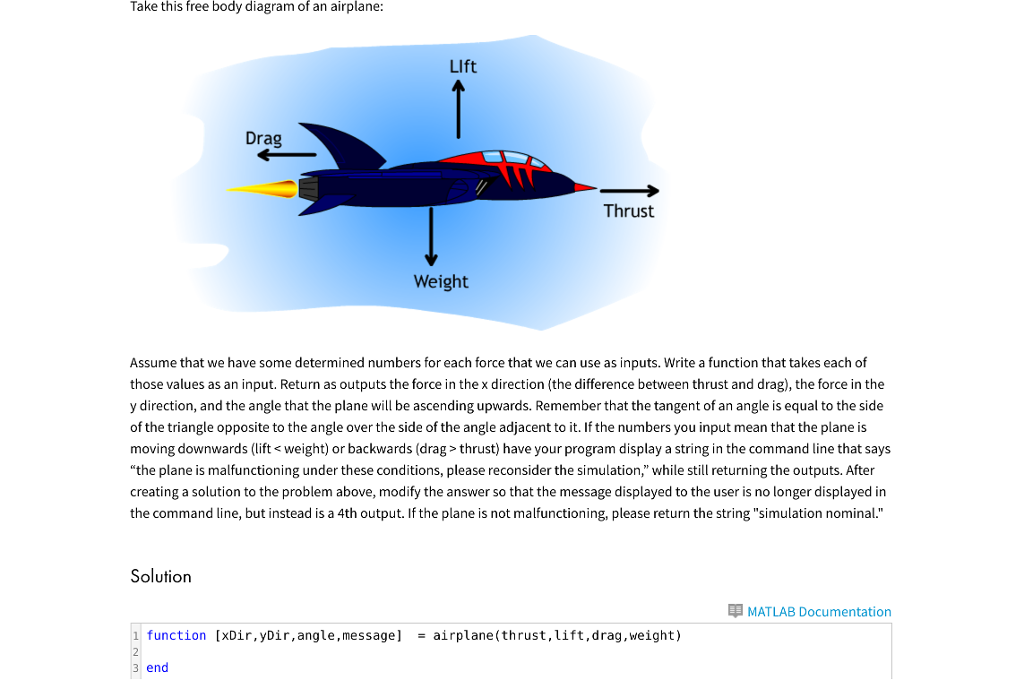# Question & Answer: Take this free body diagram of an airplane: Assume that we have some determined numbers for each force that we can use as inputs. Write a function that takes eac…..Take this free body diagram of an airplane: Assume that we have some determined numbers for each force that we can use as inputs. Write a function that takes each of those values as an input. Return as outputs the force in the x direction (the difference between thrust and drag), the force in the y direction, and the angle that the plane will be ascending upwards. Remember that the tangent of an angle is equal to the side of the triangle opposite to the angle over the side of the angle adjacent to it. If the numbers you input mean that the plane is moving downwards (lift thrust) have your program display a string in the command line that says “the plane is malfunctioning under these conditions, please reconsider the simulation, ” while still returning the outputs. After creating a solution to the problem above, modify the answer so that the message displayed to the user is no longer displayed in the command line, but instead is a 4th output. If the plane is not malfunctioning, please return the string “simulation nominal.”

Don't use plagiarized sources. Get Your Custom Essay on
Question & Answer: Take this free body diagram of an airplane: Assume that we have some determined numbers for each force that we can use as inputs. Write a function that takes eac…..
GET AN ESSAY WRITTEN FOR YOU FROM AS LOW AS \$13/PAGE

function[xDir,yDir,angle,message]=airplane(thrust,lift,drag,weight)
xDir=thrust-drag;
yDir=lift-weight;
angle=atan(yDir/xDir);
if xDir<0 || yDir<0
message=’the plane is malfunctioning under these conditions,please reconsider the simulation’;
else
message=’simulation nominal’;
end